Anzeige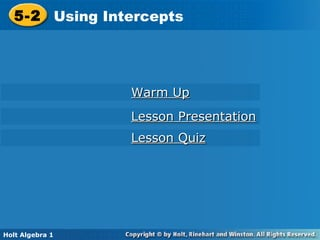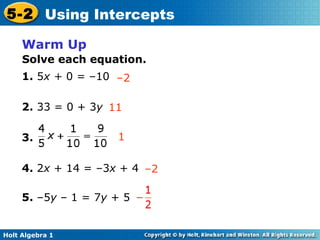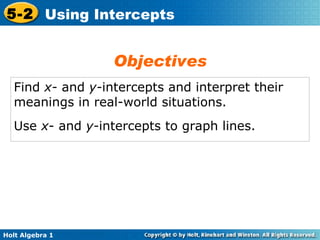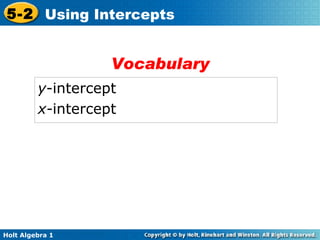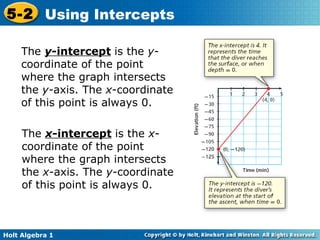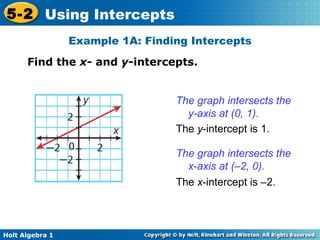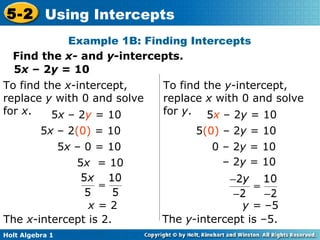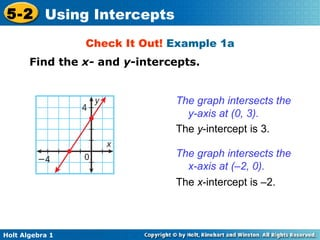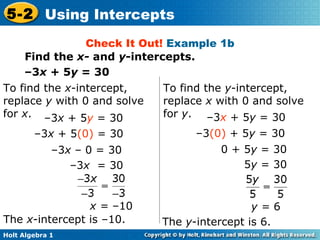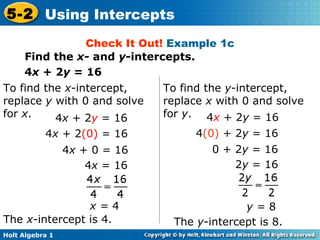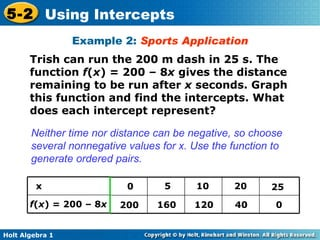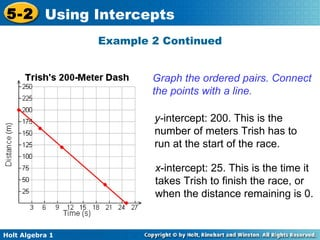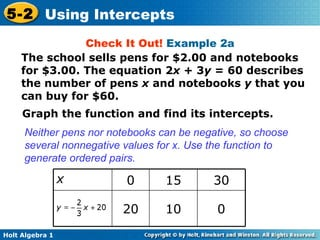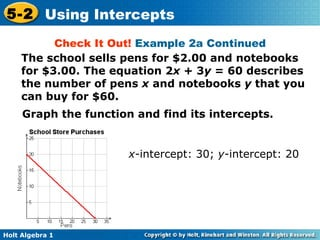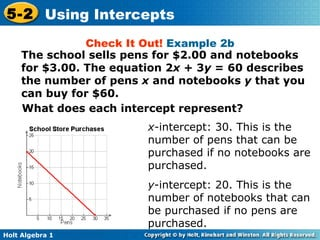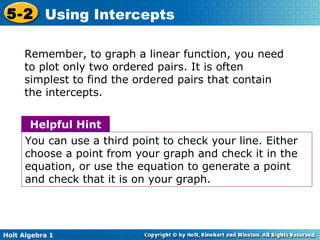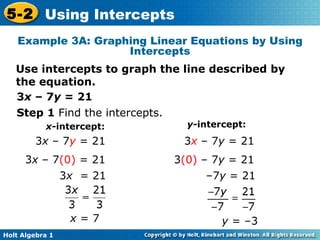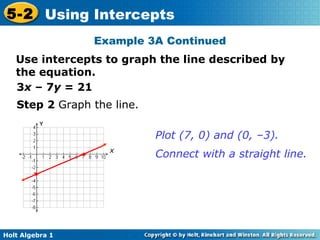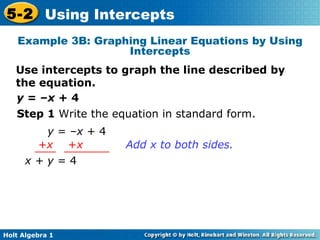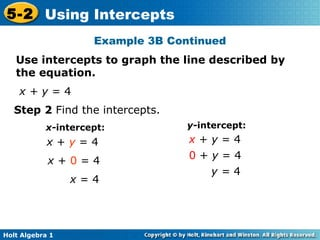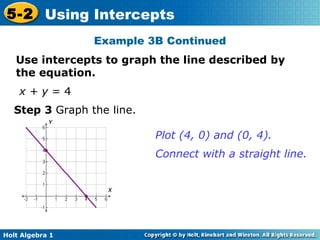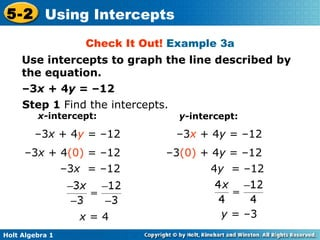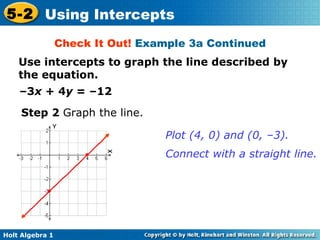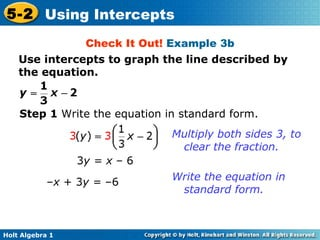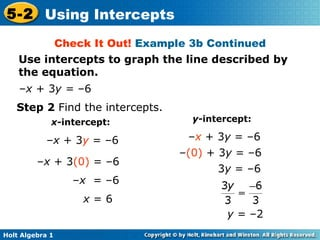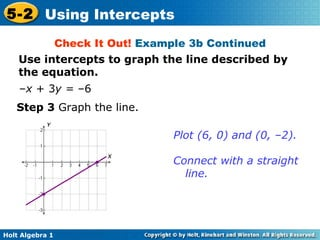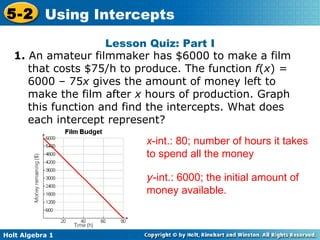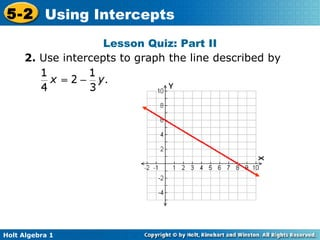1 von 28
Anzeige

### Chapter 5 Using Intercepts

1. 5-2 Using Intercepts Holt Algebra 1 Lesson Quiz Lesson Presentation Warm Up
2. Warm Up 1. 5 x + 0 = –10 Solve each equation. – 2 11 1 – 2 2. 33 = 0 + 3 y 3. 4. 2 x + 14 = –3 x + 4 5. –5 y – 1 = 7 y + 5
3. Find x- and y- intercepts and interpret their meanings in real-world situations. Use x - and y- intercepts to graph lines. Objectives
4. y -intercept x- intercept Vocabulary
5. The y -intercept is the y -coordinate of the point where the graph intersects the y- axis. The x -coordinate of this point is always 0. The x -intercept is the x -coordinate of the point where the graph intersects the x -axis. The y -coordinate of this point is always 0.
6. Example 1A: Finding Intercepts Find the x- and y -intercepts. The graph intersects the y-axis at (0, 1). The y -intercept is 1. The graph intersects the x-axis at (–2, 0). The x -intercept is –2.
7. Example 1B: Finding Intercepts 5 x – 2 y = 10 To find the y -intercept, replace x with 0 and solve for y . To find the x -intercept, replace y with 0 and solve for x . Find the x- and y -intercepts. 5 x – 2 y = 10 5 x – 2 (0) = 10 5 x – 0 = 10 5 x = 10 x = 2 The x -intercept is 2. 5 x – 2 y = 10 5 (0) – 2 y = 10 0 – 2 y = 10 – 2 y = 10 y = –5 The y -intercept is –5.
8. Check It Out! Example 1a Find the x- and y -intercepts. The graph intersects the y-axis at (0, 3). The y -intercept is 3. The graph intersects the x-axis at (–2, 0). The x -intercept is –2.
9. Check It Out! Example 1b Find the x- and y -intercepts. – 3 x + 5 y = 30 To find the y -intercept, replace x with 0 and solve for y . To find the x -intercept, replace y with 0 and solve for x . – 3 x + 5 y = 30 – 3 x + 5 (0) = 30 – 3 x – 0 = 30 – 3 x = 30 x = –10 The x -intercept is –10. – 3 x + 5 y = 30 – 3 (0) + 5 y = 30 0 + 5 y = 30 5 y = 30 y = 6 The y -intercept is 6.
10. Check It Out! Example 1c Find the x- and y -intercepts. 4 x + 2 y = 16 To find the y -intercept, replace x with 0 and solve for y . To find the x -intercept, replace y with 0 and solve for x . 4 x + 2 y = 16 4 x + 2 (0) = 16 4 x + 0 = 16 4 x = 16 x = 4 The x -intercept is 4. 4 x + 2 y = 16 4 (0) + 2 y = 16 0 + 2 y = 16 2 y = 16 y = 8 The y -intercept is 8.
11. Example 2: Sports Application Trish can run the 200 m dash in 25 s. The function f ( x ) = 200 – 8 x gives the distance remaining to be run after x seconds. Graph this function and find the intercepts. What does each intercept represent? Neither time nor distance can be negative, so choose several nonnegative values for x. Use the function to generate ordered pairs. f ( x ) = 200 – 8 x 200 25 0 5 10 20 x 160 120 40 0
12. Graph the ordered pairs. Connect the points with a line. x -intercept: 25. This is the time it takes Trish to finish the race, or when the distance remaining is 0. y- intercept: 200. This is the number of meters Trish has to run at the start of the race. Example 2 Continued
13. Check It Out! Example 2a The school sells pens for \$2.00 and notebooks for \$3.00. The equation 2 x + 3 y = 60 describes the number of pens x and notebooks y that you can buy for \$60. Graph the function and find its intercepts. Neither pens nor notebooks can be negative, so choose several nonnegative values for x. Use the function to generate ordered pairs. 0 10 20 30 15 0 x
14. Check It Out! Example 2a Continued The school sells pens for \$2.00 and notebooks for \$3.00. The equation 2 x + 3 y = 60 describes the number of pens x and notebooks y that you can buy for \$60. Graph the function and find its intercepts. x -intercept: 30; y -intercept: 20
15. Check It Out! Example 2b The school sells pens for \$2.00 and notebooks for \$3.00. The equation 2 x + 3 y = 60 describes the number of pens x and notebooks y that you can buy for \$60. x- intercept: 30. This is the number of pens that can be purchased if no notebooks are purchased. y -intercept: 20. This is the number of notebooks that can be purchased if no pens are purchased. What does each intercept represent?
16. Remember, to graph a linear function, you need to plot only two ordered pairs. It is often simplest to find the ordered pairs that contain the intercepts. Helpful Hint You can use a third point to check your line. Either choose a point from your graph and check it in the equation, or use the equation to generate a point and check that it is on your graph.
17. Example 3A: Graphing Linear Equations by Using Intercepts Use intercepts to graph the line described by the equation. 3 x – 7 y = 21 Step 1 Find the intercepts. x- intercept: y- intercept: 3 x = 21 – 7 y = 21 y = –3 3 x – 7 y = 21 3 x – 7 (0) = 21 x = 7 3 x – 7 y = 21 3 (0) – 7 y = 21
18. Step 2 Graph the line. Plot (7, 0) and (0, –3). Connect with a straight line. Example 3A Continued Use intercepts to graph the line described by the equation. 3 x – 7 y = 21 x
19. Example 3B: Graphing Linear Equations by Using Intercepts Use intercepts to graph the line described by the equation. y = – x + 4 Step 1 Write the equation in standard form. y = – x + 4 x + y = 4 Add x to both sides. +x + x
20. Example 3B Continued Use intercepts to graph the line described by the equation. Step 2 Find the intercepts. x- intercept: y- intercept: x = 4 y = 4 x + y = 4 x + y = 4 x + 0 = 4 x + y = 4 0 + y = 4
21. Example 3B Continued Use intercepts to graph the line described by the equation. Step 3 Graph the line. x + y = 4 Plot (4, 0) and (0, 4). Connect with a straight line.
22. Use intercepts to graph the line described by the equation. – 3 x + 4 y = –12 Check It Out! Example 3a x = 4 y = –3 Step 1 Find the intercepts. x- intercept: y- intercept: – 3 x + 4 y = –12 – 3 x + 4 (0) = –12 – 3 x = –12 – 3 x + 4 y = –12 – 3 (0) + 4 y = –12 4 y = –12
23. Use intercepts to graph the line described by the equation. – 3 x + 4 y = –12 Check It Out! Example 3a Continued Step 2 Graph the line. Plot (4, 0) and (0, –3). Connect with a straight line.
24. Use intercepts to graph the line described by the equation. Step 1 Write the equation in standard form. Check It Out! Example 3b 3 y = x – 6 – x + 3 y = –6 Multiply both sides 3, to clear the fraction. Write the equation in standard form.
25. Step 2 Find the intercepts. x- intercept: y- intercept: – x + 3 y = –6 – x + 3 (0) = –6 – x = –6 x = 6 – x + 3 y = –6 – (0) + 3 y = –6 3 y = –6 y = –2 Use intercepts to graph the line described by the equation. Check It Out! Example 3b Continued – x + 3 y = –6
26. Check It Out! Example 3b Continued Step 3 Graph the line. Plot (6, 0) and (0, –2). Connect with a straight line. Use intercepts to graph the line described by the equation. – x + 3 y = –6
27. Lesson Quiz: Part I 1. An amateur filmmaker has \$6000 to make a film that costs \$75/h to produce. The function f ( x ) = 6000 – 75 x gives the amount of money left to make the film after x hours of production. Graph this function and find the intercepts. What does each intercept represent? x -int.: 80; number of hours it takes to spend all the money y -int.: 6000; the initial amount of money available.
28. Lesson Quiz: Part II 2. Use intercepts to graph the line described by
Anzeige